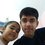# TWO OF MY FORMULAS !!!!!!!!!!!!!!!!!!!

Hello, my dear brilliant members. Well, I wanted to give you some simple challenges. These are 2 of my new formulas which are (A)Finding the height of any triangle with sides a,b,c in terms of a, b and c. Obviously, there can be 3 heights but you find for base b. (B) Finding the length of median of any triangle of side a, b, c in terms of a, b and c and the median should be bisecting side b. Well, it is extremely easy and the challenge is that you find these formulas with proper proofs. Don't Google it as I am pretty sure you all can do it. Thank you and everyone comment on this, try and find the formulas yourself and post it in the comments.Note by Kushagra Sahni
6 years, 4 months ago

This discussion board is a place to discuss our Daily Challenges and the math and science related to those challenges. Explanations are more than just a solution — they should explain the steps and thinking strategies that you used to obtain the solution. Comments should further the discussion of math and science.

When posting on Brilliant:

• Use the emojis to react to an explanation, whether you're congratulating a job well done , or just really confused .
• Ask specific questions about the challenge or the steps in somebody's explanation. Well-posed questions can add a lot to the discussion, but posting "I don't understand!" doesn't help anyone.
• Try to contribute something new to the discussion, whether it is an extension, generalization or other idea related to the challenge.

MarkdownAppears as
*italics* or _italics_ italics
**bold** or __bold__ bold
- bulleted- list
• bulleted
• list
1. numbered2. list
1. numbered
2. list
Note: you must add a full line of space before and after lists for them to show up correctly
paragraph 1paragraph 2

paragraph 1

paragraph 2

[example link](https://brilliant.org)example link
> This is a quote
This is a quote
    # I indented these lines
# 4 spaces, and now they show
# up as a code block.

print "hello world"
# I indented these lines
# 4 spaces, and now they show
# up as a code block.

print "hello world"
MathAppears as
Remember to wrap math in $$ ... $$ or $ ... $ to ensure proper formatting.
2 \times 3 $2 \times 3$
2^{34} $2^{34}$
a_{i-1} $a_{i-1}$
\frac{2}{3} $\frac{2}{3}$
\sqrt{2} $\sqrt{2}$
\sum_{i=1}^3 $\sum_{i=1}^3$
\sin \theta $\sin \theta$
\boxed{123} $\boxed{123}$

Sort by:

u suck

- 5 years, 2 months ago

Proofs: (A) Take $\triangle ABC$ with an altitude $BD$ of length $h$ perpendicular to $b$. Let $DC=x$, and thus, $AD=b-x$. Now, by Pythagoras Theorem, $a^{2}-x^{2}=c^{2}-(b-x)^{2}$ $\Rightarrow$ $a^{2}+b^{2}-c^{2}=2bx$ $\Rightarrow$ $x=\frac{a^{2}+b^{2}-c^{2}}{2b}$

Also, $x^{2}+h^{2}=a^{2}$ $\Rightarrow$ $h^{2}=a^{2}+x^{2}$

Substituting for $x$ into the first equation, $h^{2}=c^{2}-(b-\frac{a^{2}+b^{2}+c^{2}}{2b})^{2}$

By simplifying and bringing to the same denominator, we get $h=\sqrt{\frac{2b^2c^2 + 2c^2b^2 + 2a^2b^2-a^4-b^4-c^4}{4b^2}}$ $\Rightarrow$ $h=\frac{\sqrt{2b^2c^2+2c^2a^2+2a^2b^2-a^4-b^4-c^4}}{2b}$, which can be simplified, as shown in my other comment.

- 6 years, 4 months ago

It still can be simplified. Apply the identity a^2-b^2

- 6 years, 4 months ago

And yes. Post a proof for median one as well

- 6 years, 4 months ago

I have gotten both formulae.

(A) $h_b = \frac{\sqrt{2a^{2}b^{2}+2b^{2}c^{2}+2c^{2}a^{2}-a^{4}-b^{4}-c^{4}}}{2b}$, where $h_b$ denotes the altitude incident on $b$

(B) $x_b = \frac{\sqrt{2a^{2}+2c^{2}-b^{2}}}{2}$, where $x_b$ denotes the median incident on $b$

Should I post a proof?

- 6 years, 4 months ago

The altitude formula can be simplified to $h_b=\frac{\sqrt{(a^{2}+b^{2}+c^{2})^{2}-4(a^{2}b^{2}+b^{2}c^{2}+c^{2}a^{2})}}{2b}$

- 6 years, 4 months ago

- 6 years, 4 months ago

Sorry, been caught up with IIT classes

- 6 years, 4 months ago

- 6 years, 4 months ago

AND YES, ALSO TELL ME THAT ARE THESE GOOD DISCOVERIES OR VERY EASY ONES ?

- 6 years, 4 months ago

YOUR MEDIAN ONE IS CORRECT, BUT THE ALTITUDE ONE CAN BE SIMPLIFIED FURTHER. SIMPLIFY IT USING SUITABLE IDENTITIES AND THEN POST A PROOF FOR BOTH

- 6 years, 4 months ago